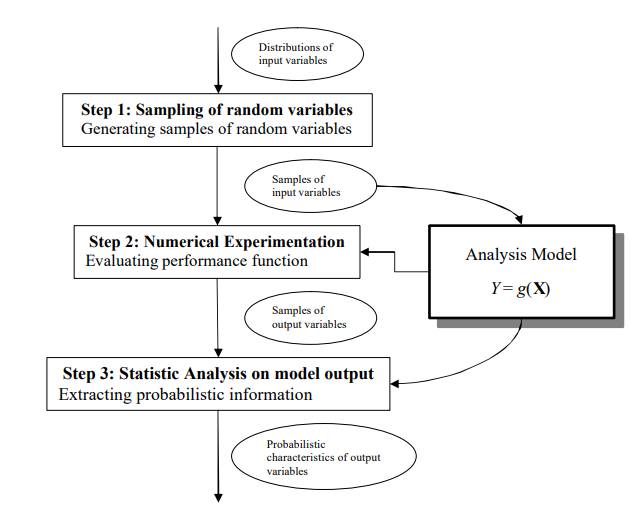### Definition

Monte Carlo Simulation is a problem solving technique used to approximate the probability of certain outcomes by running multiple trials, called simulations, using random variables.

### More details

Monte Carlo Simulation is a method of probability analysis done by running a number of variables through a model in order to determine the different outcomes.

By using Monte Carlo Simulation, decision makers are able to determine the range of possibilities and the current probability for any choice of actions.

The Monte Carlo Simulation is named for the city Monte Carlo in Monaco, which is known for its casinos. Casinos have lot of games of chance (Gambling games) like roulette, dice, and slot machines, exhibit random behavior.A Monte Carlo simulation works by constructing a mathematical model of decision under consideration. Then, the simulation is run under any aspects of that model. Different random variables are also input into this model until there are no other outcomes to be plotted in the probability distribution curve. This is usually done on computers because Monte Carlo simulation can be run tens of thousands of times in order to cover all possible outcomes.

Based on the shape of the probability distribution curve created by the Monte Carlo simulation, decision makers will know the utilmate decision fall within a various of probabilities. They then take the decision based on the risk they willing to take to get the outcome they want or need.